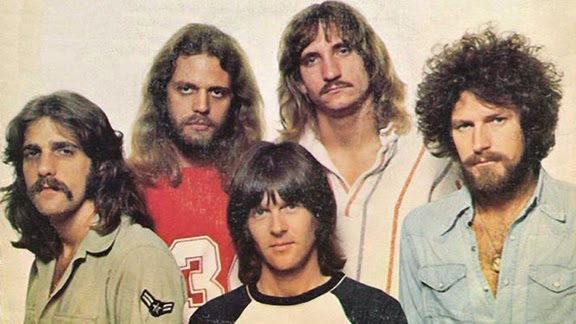## Monday, July 28, 2014

### 33 | The Eagles, from Don Henley to to Don FelderThe = 20+8+5 = 33 | Eagles = 5+1+7+12+5+19 = 49
The Eagles formed in 1971 and became the most successful commercial band of the decade; even today they're still successfully touring.  In 1971 they began with members Glenn Frey, Don Henley, Bernie Leadon and Randy Meisner.  From 1974 to 2001, the band also featured the guitar work of Don Felder.  Let us look at the Gematria of these names.
• Glenn = 7+3+5+5+5 = 25
• Frey = 6+9+5+7 = 27
• Don = 4+15+14 = 33
• Henley = 8+5+5+3+5+7 = 33
• Bernie = 2+5+9+5+9+5 = 35
• Leadon = 3+5+1+4+6+5 = 24
• Randy = 9+1+5+4+7 = 26
• Meisner = 4+5+9+1+5+5+9 = 38
• Don = 4+15+14 = 33
• Felder = 6+5+3+4+5+9 = 32
If the Eagles were Destiny's Child, Don Henley would be Beyonce.
• Destiny = 4+5+1+2+9+5+7 = 33
• Beyonce = 2+5+7+6+5+3+5 = 33
Glenn Frey was born November 6, 1948
• 11/6/1948 = 1+1+6+1+9+4+8 = 30
Don Henley was born July 22, 1947
• 7/22/1947 = 7+2+2+1+9+4+7 = 32
Bernie Leadon was born July 19, 1947
• 7/19/1947 = 7+1+9+1+9+4+7 = 38
Randy Meisner was born March 8, 1946
• 3/8/1946 = 3+8+1+9+4+6 = 31
Don Felder was born September 21, 1947
• 9/21/1947 = 9+2+1+1+9+4+7 = 33
In the case of the Eagles, the Gematria of Don Henley and Don Felder stand out among the band members, past and present.  In the case of Don Felder, not only does he have the "33" name, he has the "33" life number.  His joining of the band in 1974 matches the numerology of his 1947 birth year.

Let us close with this thought, "The Dude", also known as "The Big Lebowski", really does not like The Eagles.
• Lebowski = 3+5+2+6+5+1+2+9 = 33

#### 1 comment:

1.AAHHHH so you are saying in a rather 'cloaked fashion' that Big Al aka Alex Lifeson does NOT like the Eagles?